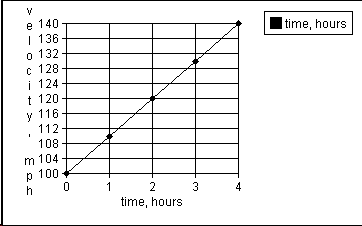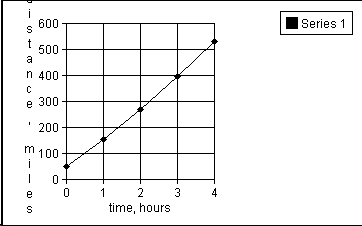+ Text Only Site
+ Non-Flash Version
+ Contact GlennVelocity and Distance for Constant Force Answers Graph the equation v = at + v0 over the domain of 0 < t < 4 hours where a= 10 mph2 and v0= 100 mph.What does the velocity intercept represent? The initial velocity of 100 mph. Calculate the slope of the graph. 10 mph2 What does the slope represent? Acceleration. What is your velocity after four hours?140 mph Does this make sense?Yes What happens to your graph if v0 = 0? The entire graph (straight line) shifts downward to a parallel line whose velocity intercept is zero. What effect does a change in acceleration a have upon your graph? It changes the slope. Using the equation x = 1/2 at2 + v0t + x0 with an x0 =50 miles, graph x over the domain of 0 < t < 4 hours.Is this a reasonable domain for this equation? The force will not remain constant for an appreciable time because lift and drag depend upon the square of the velocity. Although our acceleration is relatively small, we are changing the velocity a significant amount in four hours so we probably have assumed too large a domain.

Related Pages:
Standards
Activity
Worksheet
Lesson Index
Aerodynamics Index+ Inspector General Hotline + Equal Employment Opportunity Data Posted Pursuant to the No Fear Act + Budgets, Strategic Plans and Accountability Reports + Freedom of Information Act + The President's Management Agenda + NASA Privacy Statement, Disclaimer, and Accessibility CertificationEditor: Tom Benson NASA Official: Tom Benson Last Updated: Thu, May 13 02:38:25 PM EDT 2021 + Contact Glenn